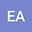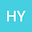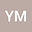Recovery of unknown coefficients in a two-dimensional hyperbolic equation with additional conditions
•••He Yang
Augusta University
Author ProfileYashar Mehraliyev
Baku State University
Author Profile## Abstract

In this paper, a nonlocal inverse boundary value problem for a two-dimensional hyperbolic equation with overdetermination conditions is studied. To investigate the solvability of the original problem, we first consider an auxiliary inverse boundary value problem and prove its equivalence (in a certain sense) to the original problem. Then using the Fourier method, solving an equivalent problem is reduced to solving a system of integral equations and by the contraction mappings principle the existence and uniqueness theorem for auxiliary problem is proved. Further, on the basis of the equivalency of these problems the uniquely existence theorem for the classical solution of the considered inverse problem is proved and some considerations on the numerical solution for this inverse problem are presented with the examples.
20 Jul 2021Submitted to Mathematical Methods in the Applied Sciences
21 Jul 2021Submission Checks Completed
21 Jul 2021Assigned to Editor
07 Aug 2021Reviewer(s) Assigned
06 Mar 2022Editorial Decision: Revise Minor